## An elaborate discussion on the various Components, Loss Functions and Metrics used for Super Resolution using Deep Learning.

Written by Bharath Raj with feedback from Yoni Osin.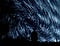Photo by Jeremy Thomas on Unsplash

# Introduction

Super Resolution is the process of recovering a High Resolution (HR) image from a given Low Resolution (LR) image. An image may have a “lower resolution” due to a smaller spatial resolution (i.e. size) or due to a result of degradation (such as blurring). We can relate the HR and LR images through the following equation: `LR = degradation(HR)`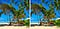A low resolution image kept besides its high resolution version. (Photo by Jarrad Horne on Unsplash)

Clearly, on applying a degradation function, we obtain the LR image from the HR image. But, can we do the inverse? In the ideal case, yes! If we know the exact degradation function, by applying its inverse to the LR image, we can recover the HR image.

But, there in lies the problem. We usually do not know the degradation function before hand. Directly estimating the inverse degradation function is an ill-posed problem. In spite of this, Deep Learning techniques have proven to be effective for Super Resolution.

This blog primarily focuses on providing an introduction to performing Super Resolution using Deep Learning by using Supervised training methods. Some important loss functions and metrics are also discussed. A lot of the content is derived from this literature review which the reader can refer to.

# Supervised Methods

As mentioned before, deep learning can be used to estimate the High Resolution (HR) image given a Low Resolution (LR) image. By using the HR image as a target (or ground-truth) and the LR image as an input, we can treat this like a supervised learning problem.

In this section, we group various deep learning approaches in the manner the convolution layers are organized. Before we move on to the groups, a primer on data preparation and types of convolutions is presented. Loss functions used to optimize the model are presented separately towards the end of this blog.

## Preparing the Data

One easy method of obtaining LR data is to degrade HR data. This is often done by blurring or adding noise. Images of lower spatial resolution can also be scaled by a classic upsampling method such as Bilinear or Bicubic interpolation. JPEG and quantization artifacts can also be introduced to degrade the image.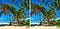Degrading a high resolution image to obtain a low resolution version of it. (Photo by Jarrad Horne on Unsplash)

One important thing to note is that it is recommended to store the HR image in an uncompressed (or lossless compressed) format. This is to prevent degradation of the quality of the HR image due to lossy compression, which may give sub-optimal performance.

## Types of Convolutions

Besides classic 2D Convolutions, several interesting variants can be used in networks for improved results. Dilated (Atrous) convolutions can provide a greater effective field of view, hence using information that are separated by a large distance. Skip connections, Spatial Pyramid Pooling and Dense Blocks motivate combining both low level and high level features to enhance performance.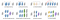Network design strategies. (Source)

The above image mentions a number of network design strategies. You can refer to this paper for more information. For a primer on the different types of convolutions commonly used in deep learning, you may refer to this blog.

## Group 1 — Pre-Upsampling

In this method, the low resolution images are first interpolated to obtain a “coarse” high resolution image. Now, CNNs are used to learn an end-to-end mapping from the interpolated low resolution images to the high resolution images. The intuition was that it may be easier to first upsample the low-resolution images using traditional methods (such as Bilinear interpolation) and then refine the resultant than learn a direct mapping from a low-dimensional space to a high-dimensional space.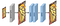A typical pre-upsampling network. (Source)

You can refer to page 5 of this paper for some models using this technique. The advantage is that since the upsampling is handled by traditional methods, the CNN only needs to learn how to refine the coarse image, which is simpler. Moreover, since we are not using transposed convolutions here, checkerboard artifacts maybe circumvented. However the downside is that the predefined upsampling methods may amplify noise and cause blurring.

## Group 2— Post-Upsampling

In this case the low resolution images are passed to the CNNs as such. Upsampling is performed in the last layer using a learnable layer.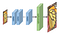A typical post-upsampling network. (Source)

The advantage of this method is that feature extraction is performed in the lower dimensional space (before upsampling) and hence the computational complexity is reduced. Furthermore, by using an learnable upsampling layer, the model can be trained end-to-end.

## Group 3— Progressive Upsampling

In the above group, even though the computational complexity was reduced, only a single upsampling convolution was used. This makes the learning process harder for large scaling factors. To address this drawback, a progressive upsampling framework was adopted by works such as Laplacian Pyramid SR Network (LapSRN) and Progressive SR (ProSR). The models in this case use a cascade of CNNs to progressively reconstruct high resolution images at smaller scaling factors at each step.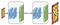A typical progressive-upsampling network. (Source)

By decomposing a difficult task into simpler tasks, the learning difficulty is greatly reduced and better performance can be obtained. Moreover, learning strategies like curriculum learning can be integrated to further reduce learning difficulty and improve final performance.

## Group 4 — Iterative Up and Down Sampling

Another popular model architecture is the hourglass (or U-Net) structure. Some variants such as the Stacked Hourglass network use several hourglass structures in series, effectively alternating between the process of upsampling and downsampling.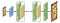A typical iterative up-and-down sampling network. (Source)

The models under this framework can better mine the deep relations between the LR-HR image pairs and thus provide higher quality reconstruction results.

# Loss Functions

Loss functions are used to measure the difference between the generated High Resolution image and the ground truth High Resolution image. This difference (error) is then used to optimize the supervised learning model. Several classes of loss functions exist where each of which penalize a different aspect of the generated image.

Often, more than one loss function is used by weighting and summing up the errors obtained from each loss function individually. This enables the model to focus on aspects contributed by multiple loss functions simultaneously.

`total_loss = weight_1 * loss_1 + weight_ 2 * loss_2 + weight_3 * loss_3`

In this section we will explore some popular classes of loss functions used for training the models.

## Pixel Loss

Pixel-wise loss is the simplest class of loss functions where each pixel in the generated image is directly compared with each pixel in the ground-truth image. Popular loss functions such as the L1 or L2 loss or advanced variants such as the Smooth L1 loss are used.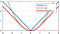Plot of Smooth L1 Loss. (Source)

The PSNR metric (discussed below) is highly correlated with the pixel-wise difference, and hence minimizing the pixel loss directly maximizes the PSNR metric value (indicating good performance). However, pixel loss does not take into account the image quality and the model often outputs perceptually unsatisfying results (often lacking high frequency details).

## Content Loss

This loss evaluates the image quality based on its perceptual quality. An interesting way to do this is by comparing the high level features of the generated image and the ground truth image. We can obtain these high level features by passing both of these images through a pre-trained image classification network (such as a VGG-Net or a ResNet).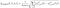Content loss between a ground truth image and a generated image. (Source)

The equation above calculates the content loss between a ground-truth image and a generated image, given a pre-trained network (Φ) and a layer (l) of this pre-trained network at which the loss is computed. This loss encourages the generated image to be perceptually similar to the ground-truth image. For this reason, it is also known as the Perceptual loss.

## Texture Loss

To enable the generated image to have the same style (texture, color, contrast etc.) as the ground truth image, texture loss (or style reconstruction loss) is used. The texture of an image, as described by Gatys et. al, is defined as the correlation between different feature channels. The feature channels are usually obtained from a feature map extracted using a pre-trained image classification network (Φ).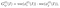Computing the Gram Matrix. (Source)

The correlation between the feature maps is represented by the Gram matrix (G), which is the inner product between the vectorized feature maps `i` and `j` on layer `l` (shown above). Once the Gram matrix is calculated for both images, calculating the texture loss is straight-forward, as shown below: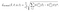Computing the Texture Loss. (Source)

By using this loss, the model is motivated to create realistic textures and visually more satisfying results.

## Total Variation Loss

The Total Variation (TV) loss is used to suppress noise in the generated images. It takes the sum of the absolute differences between neighboring pixels and measures how much noise is in the image. For a generated image, the TV loss is calculated as shown below: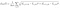Total Variation Loss used on a generated High Resolution image. (Source)

Here, `i,j,k` iterates over the height, width and channels respectively.

## Adversarial Loss

Generative Adversarial Networks (GANs) have been increasingly used for several image based applications including Super Resolution. GANs typically consist of a system of two neural networks — the Generator and the Discriminator — dueling each other.

Given a set of target samples, the Generator tries to produce samples that can fool the Discriminator into believing they are real. The Discriminator tries to resolve real (target) samples from fake (generated) samples. Using this iterative training approach, we eventually end up with a Generator that is really good at generating samples similar to the target samples. The following image shows the structure of a typical GAN.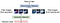GANs in action. (Source)

Advances to the basic GAN architecture were introduced for improved performance. For instance, Park et. al. used a feature-level discriminator to capture more meaningful potential attributes of real High Resolution images. You can checkout this blog for a more elaborate survey about the advances in GANs.

Typically, models trained with adversarial loss have better perceptual quality even though they might lose out on PSNR compared to those trained on pixel loss. One minor downside is that, the training process of GANs is a bit difficult and unstable. However, methods to stabilize GAN training are actively worked upon.

# Metrics

One big question is how do we quantitatively evaluate the performance of our model. A number of Image Quality Assessment (IQA) techniques (or metrics) are used for the same. These metrics can be broadly classified into two categories — Subjective metrics and Objective metrics.

Subjective metrics are based on the human observer’s perceptual evaluation whereas objective metrics are based on computational models that try to assess the image quality. Subjective metrics are often more “perceptually accurate”, however some of these metrics are inconvenient, time-consuming or expensive to compute. Another issue is that these two categories of metrics may not be consistent with each other. Hence, researchers often display results using metrics from both categories.

In this section, we will briefly explore a couple of the widely used metrics to evaluate the performance of our super resolution model.

## PSNR

Peak Signal-to-Noise Ratio (PSNR) is commonly used objective metric to measure the reconstruction quality of a lossy transformation. PSNR is inversely proportional to the logarithm of the Mean Squared Error (MSE) between the ground truth image and the generated image.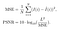Calculation of PSNR. (Source)

In the above formula, L is the maximum possible pixel value (for 8-bit RGB images, it is 255). Unsurprisingly, since PSNR only cares about the difference between the pixel values, it does not represent perceptual quality that well.

## SSIM

Structural Similarity (SSIM) is a subjective metric used for measuring the structural similarity between images, based on three relatively independent comparisons, namely luminance, contrast, and structure. Abstractly, the SSIM formula can be shown as a weighted product of the comparison of luminance, contrast and structure computed independently.SSIM is a weighted product of comparisons as described above. (Source)

In the above formula, alpha, beta and gamma are the weights of the luminance, contrast and structure comparison functions respectively. The commonly used representation of the SSIM formula is as shown below: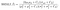Commonly used representation of the SSIM formula. (Source)

In the above formula `μ(I)`represents the mean of a particular image, `σ(I)` represents the standard deviation of a particular image,`σ(I,I’)`represents the covariance between two images, and `C1, C2` are constants set for avoiding instability. For brevity, the significance of the terms and the exact derivation is not explained in this blog and the interested reader can checkout Section 2.3.2 in this paper.

Due to the possible unevenly distribution of image statistical features or distortions, assessing image quality locally is more reliable than applying it globally. Mean SSIM (MSSIM), which splits the image into multiple windows and averages the SSIM obtained at each window, is one such method of assessing quality locally.

In any case, since SSIM evaluates the reconstruction quality from the perspective of the Human Visual System, it better meets the requirements of the perceptual assessment.

## Other IQA Scores

Without explanation, some other methods of assessing image quality are listed below. The interested reader can refer to this paper for more details.

• Mean Opinion Score (MOS)
• Task-based Evaluation
• Information Fidelity Criterion (IFC)
• Visual Information Fidelity (VIF)

# Conclusion

This blog article covered some introductory material and procedures for training deep learning models for Super Resolution. There are indeed more advanced techniques introduced by state of the art research which may yield better performance. Furthermore, researching on avenues such as unsupervised super resolution, better normalization techniques and better representative metrics could greatly further this field. The interested reader is encouraged to experiment with their innovative ideas by participating in challenges such as the PIRM Challenge.

Written by

## BeyondMinds

#### A Research Company in AI

Welcome to a place where words matter. On Medium, smart voices and original ideas take center stage - with no ads in sight. Watch
Follow all the topics you care about, and we’ll deliver the best stories for you to your homepage and inbox. Explore
Get unlimited access to the best stories on Medium — and support writers while you’re at it. Just \$5/month. Upgrade